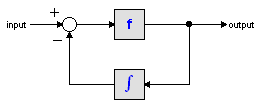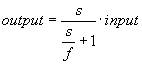﻿ 20-sim webhelp > Library > Iconic Diagrams > Mechanical > Rotation > Sensors > accelerationsensor-absolute

# accelerationsensor-absolute

Navigation:  Library > Iconic Diagrams > Mechanical > Rotation > Sensors >

# accelerationsensor-absolute## Library

Iconic Diagrams\Mechanical\Rotation\Sensors

## Use

Domains: Continuous. Size: 1-D. Kind: Iconic Diagrams (Rotation), Block Diagrams.

## Description

This model describes an acceleration sensor which derives an angular acceleration output out of a port angular velocity by differentiation. Differentiation is performed by a state variable filter:The S-domain function of this filter is equal to:where f is the cut-off frequency. For very high values of f, the output becomes the pure derivative of the input. High values of f, however, increase simulations times. A good trade-off is a starting value of 1e5.

The equations of this model are:

alpha = d( p.omega)/dt;

p.T = indifferent;

## Interface

 Ports Description p Rotation port p. Causality fixed force out Output alpha Absolute angular acceleration [rad/s2] Parameters f alpha_initial Cut-off frequency of the differentiation [Hz]. Initial angular acceleration [m/s].